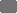# gms | German Medical Science

## GMDS 2013: 58. Jahrestagung der Deutschen Gesellschaft für Medizinische Informatik, Biometrie und Epidemiologie e. V. (GMDS)

Deutsche Gesellschaft für Medizinische Informatik, Biometrie und Epidemiologie

01. - 05.09.2013, Lübeck

## Extension of a semi-classical signal analysis method

Meeting Abstract

### Search Medline for

•Thomas Schanze - Technische Hochschule Mittelhessen (THM), Giessen, DE

GMDS 2013. 58. Jahrestagung der Deutschen Gesellschaft für Medizinische Informatik, Biometrie und Epidemiologie e.V. (GMDS). Lübeck, 01.-05.09.2013. Düsseldorf: German Medical Science GMS Publishing House; 2013. DocAbstr.328

Published: August 27, 2013

This is an Open Access article distributed under the terms of the Creative Commons Attribution License (http://creativecommons.org/licenses/by-nc-nd/3.0/deed.en). You are free: to Share – to copy, distribute and transmit the work, provided the original author and source are credited.

### Text

Introduction: Recently a semi-classical signal analysis method has been introduced . The main idea is to interpret a signal as the potential of the time-independent Schrödinger equation. The Schrödinger operator is given by the sum of the kinetic energy operator with weighting parameter h and the potential operator. If the potential is real and belongs to the Faddeev class, then it can be expressed as the sum of squared eigenfunctions weighted with corresponding negative eigenvalues, related to the discrete spectrum of the Schrödinger operator, and an integral associated with the continuous spectrum. Moreover, the method has been extended to the noisy signal case . However, the approximation and/or noise-reduction properties of the method depend on h and on the number of the eigenterms summed up. Because of its construction, the semi-classical signal analysis method is restricted to negative potentials, i.e. signals.

Materials and Methods: We solved this restriction by using signal transformations as well as by constructing novel discrete Schrödinger operators.

Results: The obvious solution of an appropriate DC-shifting of the signal has some drawbacks with respect to method’s approximation or signal recovery properties. However, a better transformation solution is to split the original signal s in a semi-positive signal s+, which is zero when s is negative and equal to s otherwise, and a semi-negative signal s- such that the sum of s+ and s- equals s. Then the semi-classical signal analysis method can be applied to s+ and s- and the resulting two signal approximations can be combined linearly to obtain an approximation for s. However, this solution is computationally more time-consuming than the first and the following third solution. For discrete signals the Schrödinger operator is the sum of two matrices. The first matrix is a second order differentiation matrix, the second is a diagonal matrix, whose entries are given by the signal to be analyzed. Any matrix can be decomposed in a diagonal and an off-diagonal matrix. Assume that the off-diagonal second order differentiation matrix has a checkerboard pattern, such that every element on the diagonal and on every second sub- and super-diagonal is zero, then negative and positive eigenvalues and related eigenfunctions of the Schrödinger matrix can be used for signal’s approximation or recovery. In addition, we discovered that positive and negative eigenvalues are linked and developed a method to construct appropriate second order differentiation matrices of various numerical error orders. The approximation or signal recovery properties of this method depend on the properties of the second order matrix differentiation operator, on the number of eigenvalues and eigenvectors used, and on signal properties.

Discussion: We launched the extended semi-classical signal analysis method. First applications to simulated and biomedical signals indicate method’s range of use and, consequently, its potential.

### References

1.
Laleg-Kirati TM, Crépeau E, Sorine M. Semi-classical signal analysis. Mathematics of Control, Signals, and Systems (MCSS). 2012; vol Online First.
2.
Liu DY, Laleg-Kirati TM. Mathematical properties of a semi-classical signal analysis method: noisy signal case. 1st Intern Conf Sys Comp Sci; 2012.